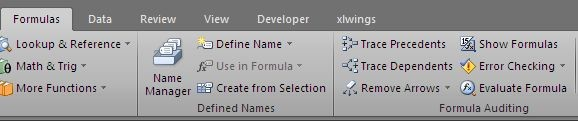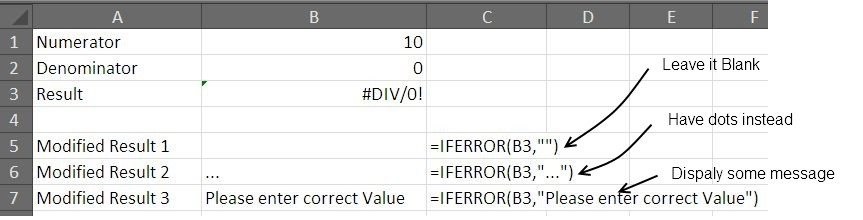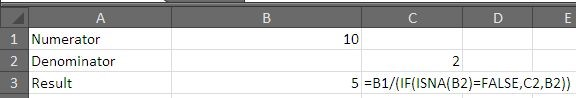# 5 Ways To Handle Or Prevent Errors In ExcelTo human is err, the same is true for excel. Whenever you are writing a formula, you are prone to commit error. It could be an error in the formula, in formula referencing, the result that formula returns and wrong formula syntax can result in an error.

If an error remains untraced, it could affect you in two ways:

1. An that does not let your formula work.

2. An error that does not stop your formula from working but gives a wrong result.

The second type of error is rather more detrimental and difficult to spot. Until unless you have produced a case where you have your results tested, such an error can make your model ineffective.

Let’s discuss how to spot errors and how to handle them:

Tools helpful in spotting and avoiding errors.

The Preventive Approach:

1- The Formula Examiner:

It is the one good thing in excel that lets you see how your formula is actually works. The feature can be accessed in Excel 2010 from Formula > Evaluate Formula in the formula auditing tools.The formula examiner can tell you that what component of your formula is not working as it is required to work.

2- Trace Precedents and Dependents.

Tracing down the input to a formula and finding to what formula or cells a value is being forwarded to is again an essential requirement for troubleshooting formulas. Trace Precedents and Dependents - feature allows you to see if the formula has correct cells as input and the value is forwarded to correct place. This comes handy for large sheets with a lot of data in it and is helpful in examine the references of the formula.

The corrective approach

3- Using IFERROR() to get desired results:

You can trap the error and get the desired result – a blank space, dots (…) or anything else you want through this formula. The syntax is simple. What ever formula you are using, that should be placed in the first argument of the formula, and the second argument contains the desired result you want to show.

In the following picture we have shows three cases if using IFERROR ().4- Using ISNA() for undesired input or providing alternative input

We can use IF() with ISNA() to check if we have desired input to a formula or not. Consider the example on Sheet2 where the cell B2 is blank and will return a Division Error. We have used and IF() with ISNA() to check for this and provided an alternative cell for input.5- Identifying Error Type with ERROR.TYPE to give Custom Messages

The Excel’s formula ERROR.TYPE give you the same as the name suggest – a value from 1-8 for different types of errors. We can have corresponding message displayed for each error. This helps in identifying as well as replying with an appropriate message for the error.